Tutorials
r programming

# Principal Component Analysis in R

In this tutorial, you'll learn how to use R PCA (Principal Component Analysis) to extract data with many variables and create visualizations to display that data.Principal Component Analysis (PCA) is a useful technique for exploratory data analysis, allowing you to better visualize the variation present in a dataset with many variables. It is particularly helpful in the case of "wide" datasets, where you have many variables for each sample. In this tutorial, you'll discover PCA in R.

More specifically, you'll tackle the following topics:

## Introduction to PCA

As you already read in the introduction, PCA is particularly handy when you're working with "wide" data sets. But why is that?

Well, in such cases, where many variables are present, you cannot easily plot the data in its raw format, making it difficult to get a sense of the trends present within. PCA allows you to see the overall "shape" of the data, identifying which samples are similar to one another and which are very different. This can enable us to identify groups of samples that are similar and work out which variables make one group different from another.

The mathematics underlying it are somewhat complex, so I won't go into too much detail, but the basics of PCA are as follows: you take a dataset with many variables, and you simplify that dataset by turning your original variables into a smaller number of "Principal Components".

But what are these exactly? Principal Components are the underlying structure in the data. They are the directions where there is the most variance, the directions where the data is most spread out. This means that we try to find the straight line that best spreads the data out when it is projected along it. This is the first principal component, the straight line that shows the most substantial variance in the data.

PCA is a type of linear transformation on a given data set that has values for a certain number of variables (coordinates) for a certain amount of spaces. This linear transformation fits this dataset to a new coordinate system in such a way that the most significant variance is found on the first coordinate, and each subsequent coordinate is orthogonal to the last and has a lesser variance. In this way, you transform a set of x correlated variables over y samples to a set of p uncorrelated principal components over the same samples.

Where many variables correlate with one another, they will all contribute strongly to the same principal component. Each principal component sums up a certain percentage of the total variation in the dataset. Where your initial variables are strongly correlated with one another, you will be able to approximate most of the complexity in your dataset with just a few principal components. As you add more principal components, you summarize more and more of the original dataset. Adding additional components makes your estimate of the total dataset more accurate, but also more unwieldy.

### Eigenvalues and Eigenvectors

Just like many things in life, eigenvectors, and eigenvalues come in pairs: every eigenvector has a corresponding eigenvalue. Simply put, an eigenvector is a direction, such as "vertical" or "45 degrees", while an eigenvalue is a number telling you how much variance there is in the data in that direction. The eigenvector with the highest eigenvalue is, therefore, the first principal component.

So wait, there are possibly more eigenvalues and eigenvectors to be found in one data set?

That's correct! The number of eigenvalues and eigenvectors that exits is equal to the number of dimensions the data set has. In the example that you saw above, there were 2 variables, so the data set was two-dimensional. That means that there are two eigenvectors and eigenvalues. Similarly, you'd find three pairs in a three-dimensional data set.

We can reframe a dataset in terms of these eigenvectors and eigenvalues without changing the underlying information. Note that reframing a dataset regarding a set of eigenvalues and eigenvectors does not entail changing the data itself, you’re just looking at it from a different angle, which should represent the data better.

Now that you've seen some of the theory behind PCA, you're ready to see all of it in action!

## A Simple PCA

In this section, you will try a PCA using a simple and easy to understand dataset. You will use the mtcars dataset, which is built into R. This dataset consists of data on 32 models of car, taken from an American motoring magazine (1974 Motor Trend magazine). For each car, you have 11 features, expressed in varying units (US units), They are as follows:

*mpg: Fuel consumption (Miles per (US) gallon): more powerful and heavier cars tend to consume more fuel.

*cyl: Number of cylinders: more powerful cars often have more cylinders

*disp: Displacement (cu.in.): the combined volume of the engine's cylinders

*hp: Gross horsepower: this is a measure of the power generated by the car

*drat: Rear axle ratio: this describes how a turn of the drive shaft corresponds to a turn of the wheels. Higher values will decrease fuel efficiency.

*wt: Weight (1000 lbs): pretty self-explanatory!

*qsec: 1/4 mile time: the cars speed and acceleration

*vs: Engine block: this denotes whether the vehicle's engine is shaped like a "V", or is a more common straight shape.

*am: Transmission: this denotes whether the car's transmission is automatic (0) or manual (1).

*gear: Number of forward gears: sports cars tend to have more gears.

*carb: Number of carburetors: associated with more powerful engines

Note that the units used vary and occupy different scales.

### Compute the Principal Components

Because PCA works best with numerical data, you'll exclude the two categorical variables (vs and am). You are left with a matrix of 9 columns and 32 rows, which you pass to the prcomp() function, assigning your output to mtcars.pca. You will also set two arguments, center and scale, to be TRUE. Then you can have a peek at your PCA object with summary().

mtcars.pca <- prcomp(mtcars[,c(1:7,10,11)], center = TRUE,scale. = TRUE)

summary(mtcars.pca)

## Importance of components:
##                           PC1    PC2     PC3     PC4     PC5     PC6
## Standard deviation     2.3782 1.4429 0.71008 0.51481 0.42797 0.35184
## Proportion of Variance 0.6284 0.2313 0.05602 0.02945 0.02035 0.01375
## Cumulative Proportion  0.6284 0.8598 0.91581 0.94525 0.96560 0.97936
##                            PC7    PC8     PC9
## Standard deviation     0.32413 0.2419 0.14896
## Proportion of Variance 0.01167 0.0065 0.00247
## Cumulative Proportion  0.99103 0.9975 1.00000


You obtain 9 principal components, which you call PC1-9. Each of these explains a percentage of the total variation in the dataset. That is to say: PC1 explains 63% of the total variance, which means that nearly two-thirds of the information in the dataset (9 variables) can be encapsulated by just that one Principal Component. PC2 explains 23% of the variance. So, by knowing the position of a sample in relation to just PC1 and PC2, you can get a very accurate view on where it stands in relation to other samples, as just PC1 and PC2 can explain 86% of the variance.

Let's call str() to have a look at your PCA object.

str(mtcars.pca)

## List of 5
##  $sdev : num [1:9] 2.378 1.443 0.71 0.515 0.428 ... ##$ rotation: num [1:9, 1:9] -0.393 0.403 0.397 0.367 -0.312 ...
##   ..- attr(*, "dimnames")=List of 2
##   .. ..$: chr [1:9] "mpg" "cyl" "disp" "hp" ... ## .. ..$ : chr [1:9] "PC1" "PC2" "PC3" "PC4" ...
##  $center : Named num [1:9] 20.09 6.19 230.72 146.69 3.6 ... ## ..- attr(*, "names")= chr [1:9] "mpg" "cyl" "disp" "hp" ... ##$ scale   : Named num [1:9] 6.027 1.786 123.939 68.563 0.535 ...
##   ..- attr(*, "names")= chr [1:9] "mpg" "cyl" "disp" "hp" ...
##  $x : num [1:32, 1:9] -0.664 -0.637 -2.3 -0.215 1.587 ... ## ..- attr(*, "dimnames")=List of 2 ## .. ..$ : chr [1:32] "Mazda RX4" "Mazda RX4 Wag" "Datsun 710" "Hornet 4 Drive" ...
##   .. ..$: chr [1:9] "PC1" "PC2" "PC3" "PC4" ... ## - attr(*, "class")= chr "prcomp"  I won't describe the results here in detail, but your PCA object contains the following information: • The center point ($center), scaling ($scale), standard deviation(sdev) of each principal component • The relationship (correlation or anticorrelation, etc) between the initial variables and the principal components ($rotation)
• The values of each sample in terms of the principal components ($x) ## Plotting PCA Now it's time to plot your PCA. You will make a biplot, which includes both the position of each sample in terms of PC1 and PC2 and also will show you how the initial variables map onto this. You will use the ggbiplot package, which offers a user-friendly and pretty function to plot biplots. A biplot is a type of plot that will allow you to visualize how the samples relate to one another in our PCA (which samples are similar and which are different) and will simultaneously reveal how each variable contributes to each principal component. Before you can get started, don't forget to first install ggbiplot! library(devtools) install_github("vqv/ggbiplot")  Next, you can call ggbiplot on your PCA: library(ggbiplot) ggbiplot(mtcars.pca)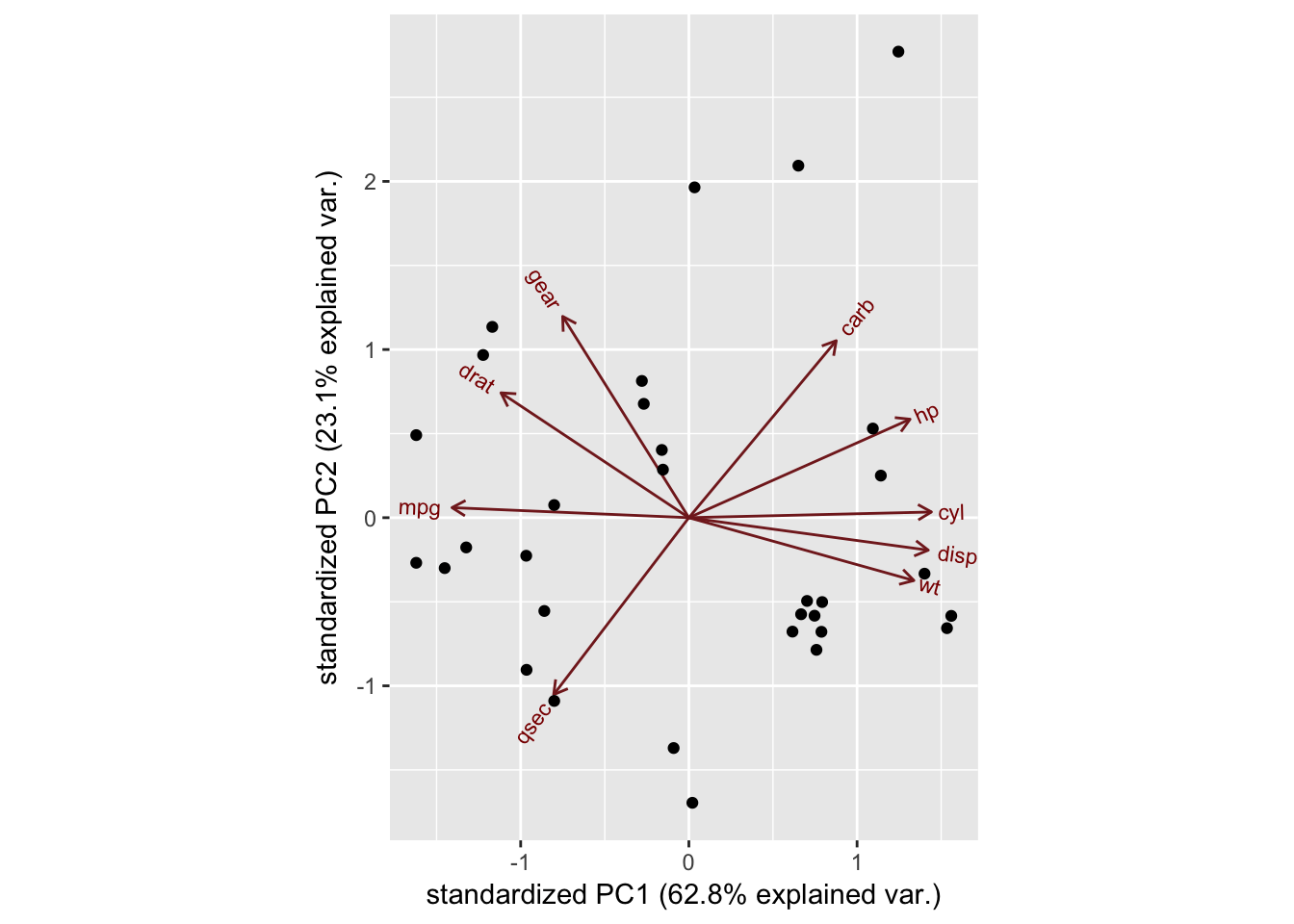The axes are seen as arrows originating from the center point. Here, you see that the variables hp, cyl, and disp all contribute to PC1, with higher values in those variables moving the samples to the right on this plot. This lets you see how the data points relate to the axes, but it's not very informative without knowing which point corresponds to which sample (car). You'll provide an argument to ggbiplot: let's give it the rownames of mtcars as labels. This will name each point with the name of the car in question: ggbiplot(mtcars.pca, labels=rownames(mtcars))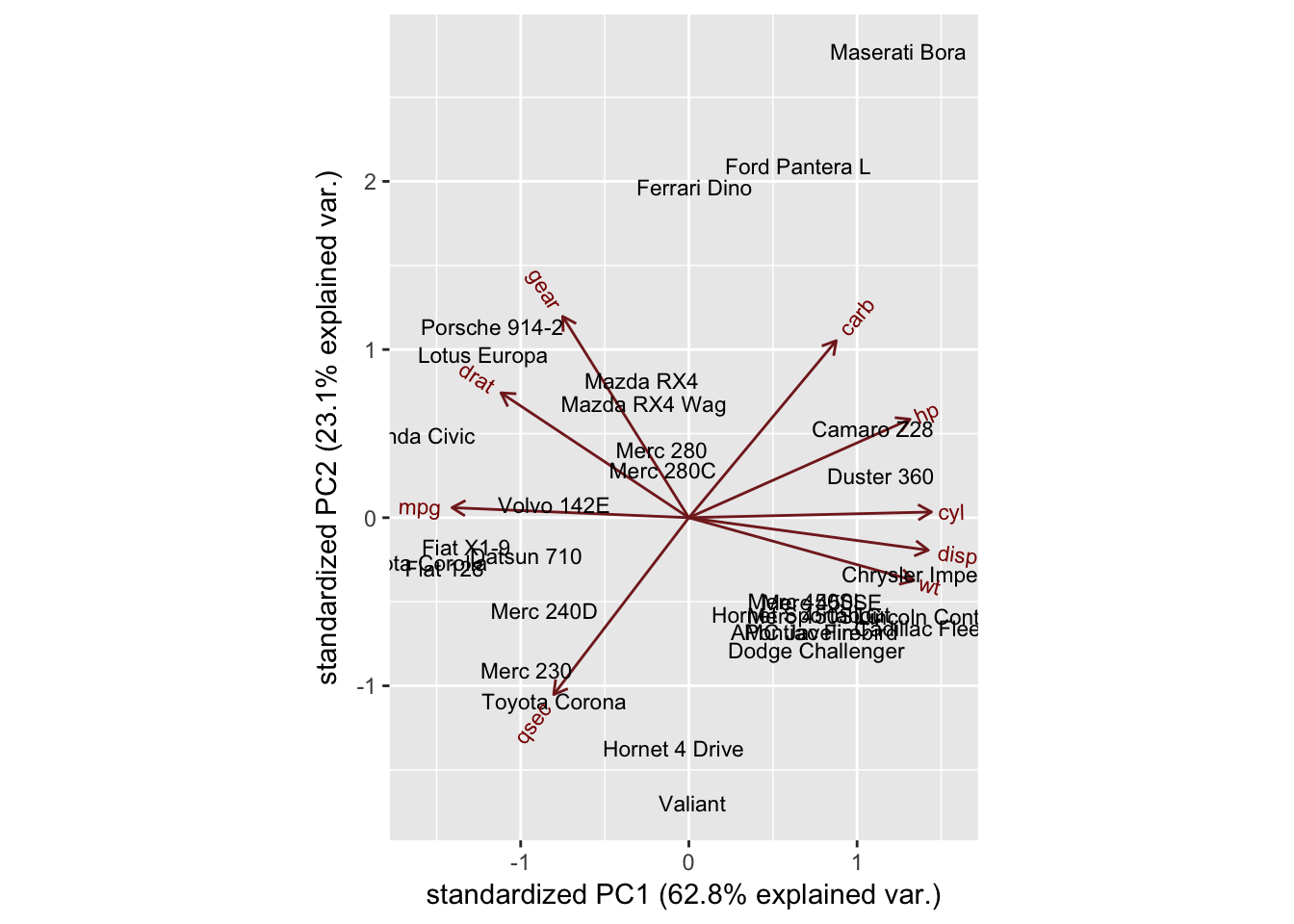Now you can see which cars are similar to one another. For example, the Maserati Bora, Ferrari Dino and Ford Pantera L all cluster together at the top. This makes sense, as all of these are sports cars. How else can you try to better understand your data? ## Interpreting the results Maybe if you look at the origin of each of the cars. You'll put them into one of three categories (cartegories?), one each for the US, Japanese and European cars. You make a list for this info, then pass it to the groups argument of ggbiplot. You'll also set the ellipse argument to be TRUE, which will draw an ellipse around each group. mtcars.country <- c(rep("Japan", 3), rep("US",4), rep("Europe", 7),rep("US",3), "Europe", rep("Japan", 3), rep("US",4), rep("Europe", 3), "US", rep("Europe", 3)) ggbiplot(mtcars.pca,ellipse=TRUE, labels=rownames(mtcars), groups=mtcars.country)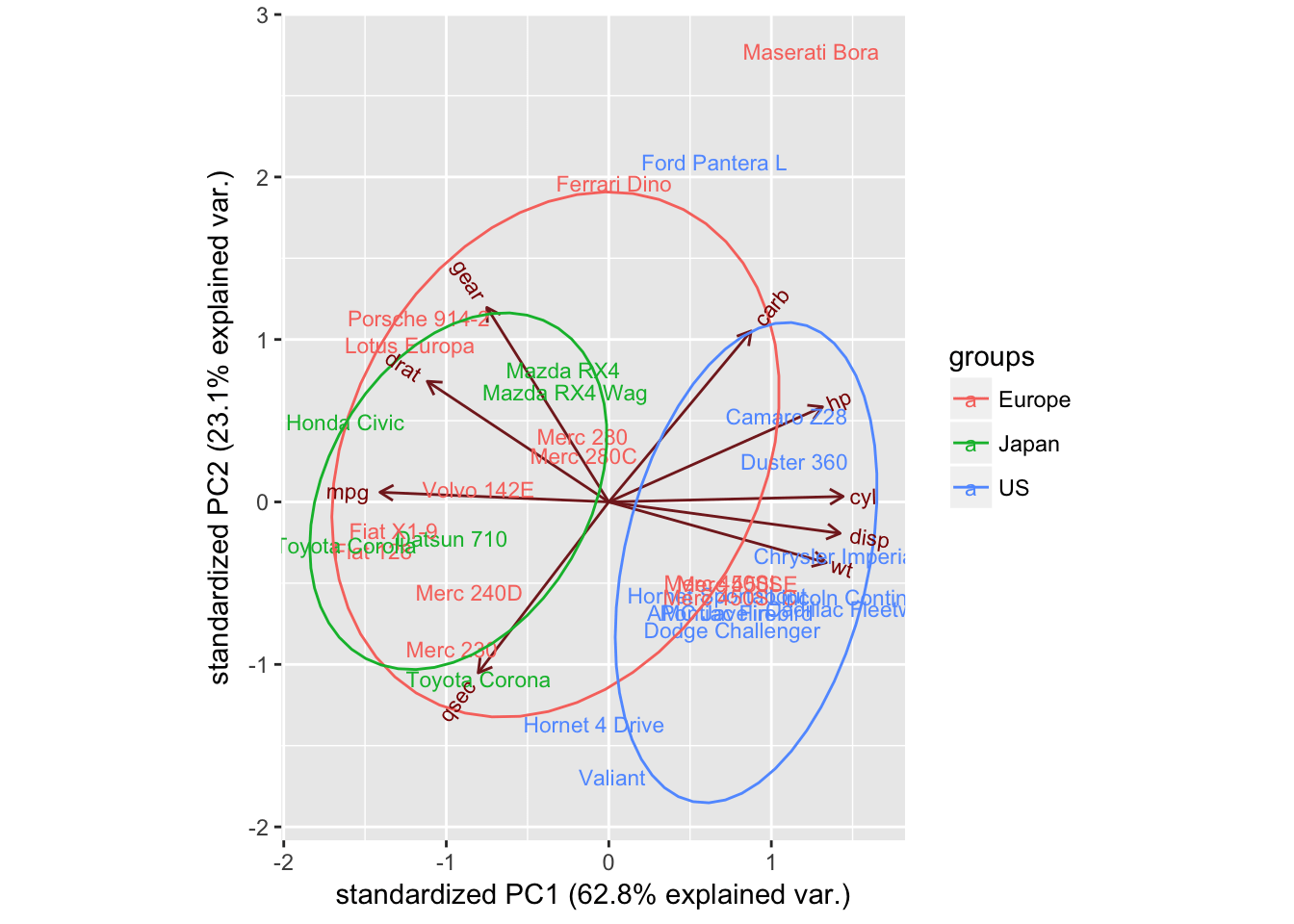Now you see something interesting: the American cars form a distinct cluster to the right. Looking at the axes, you see that the American cars are characterized by high values for cyl, disp, and wt. Japanese cars, on the other hand, are characterized by high mpg. European cars are somewhat in the middle and less tightly clustered than either group. Of course, you have many principal components available, each of which map differently to the original variables. You can also ask ggbiplot to plot these other components, by using the choices argument. Let's have a look at PC3 and PC4: ggbiplot(mtcars.pca,ellipse=TRUE,choices=c(3,4), labels=rownames(mtcars), groups=mtcars.country)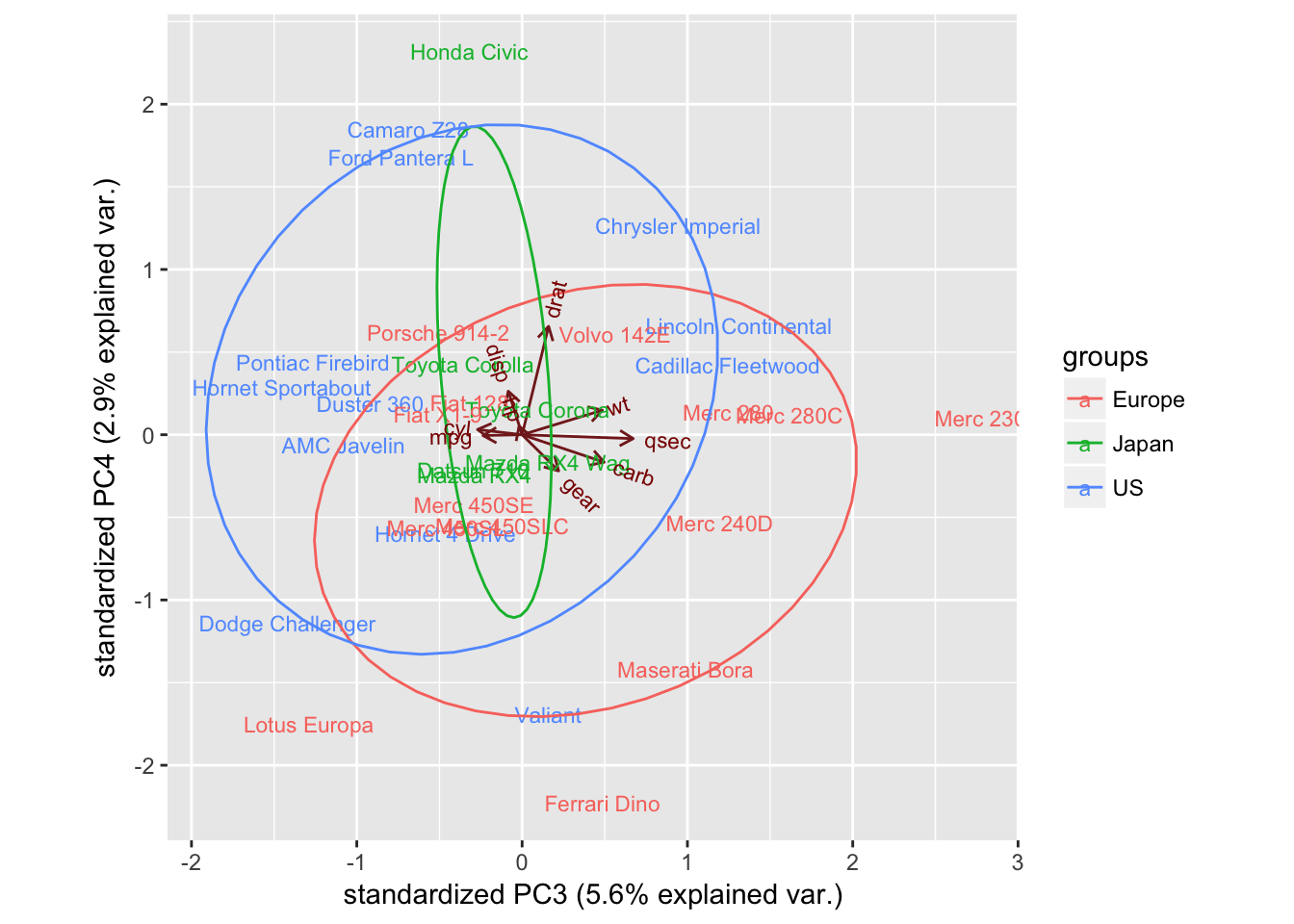You don't see much here, but this isn't too surprising. PC3 and PC4 explain very small percentages of the total variation, so it would be surprising if you found that they were very informative and separated the groups or revealed apparent patterns. Let's take a moment to recap: having performed a PCA using the mtcars dataset, we can see a clear separation between American and Japanese cars along a principal component that is closely correlated to cyl, disp, wt, and mpg. This provides us with some clues for future analyses; if we were to try to build a classification model to identify the origin of a car, these variables might be useful. ## Graphical parameters with ggbiplot There are also some other variables you can play with to alter your biplots. You can add a circle to the center of the dataset (circle argument): ggbiplot(mtcars.pca,ellipse=TRUE,circle=TRUE, labels=rownames(mtcars), groups=mtcars.country)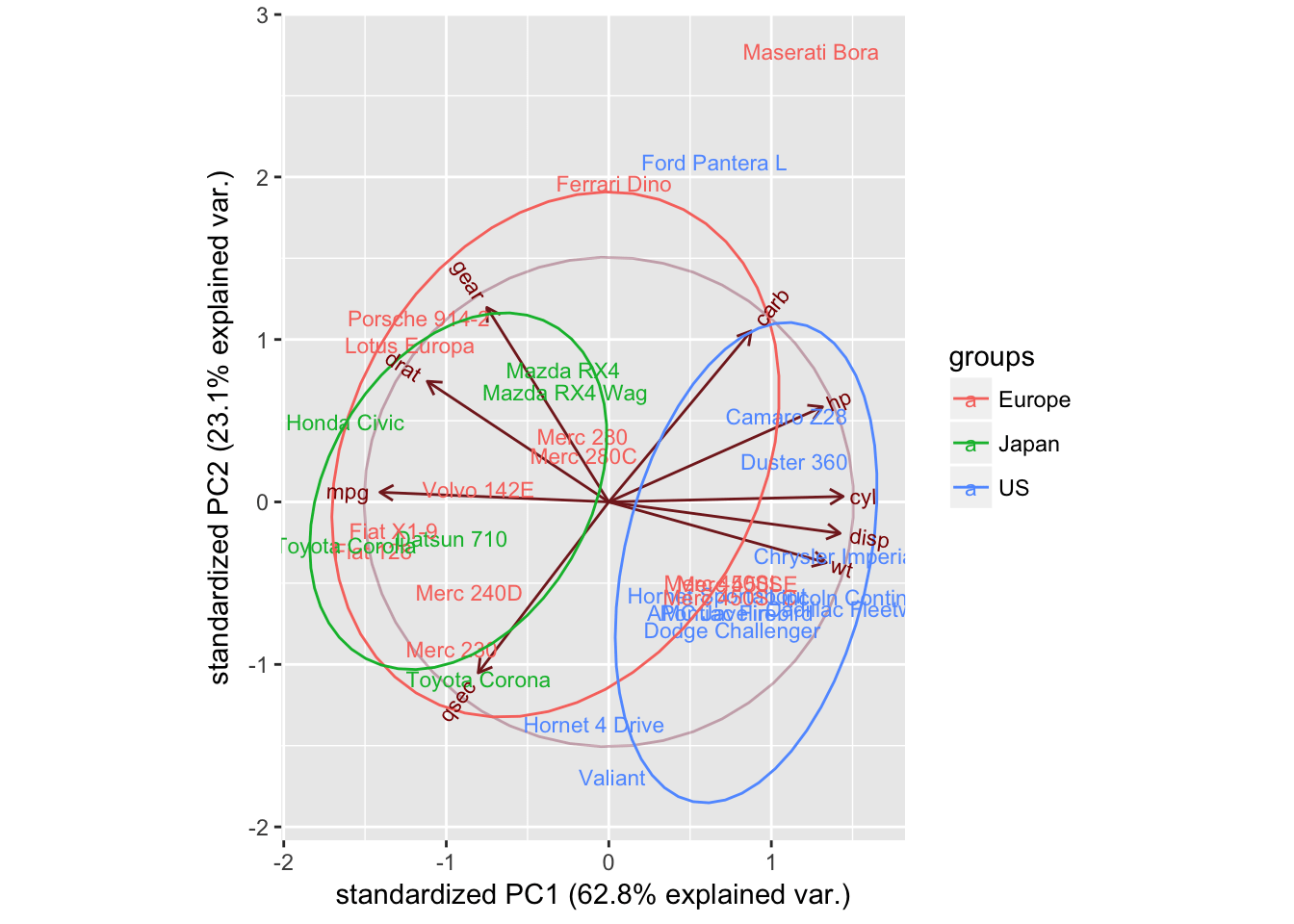You can also scale the samples (obs.scale) and the variables (var.scale): ggbiplot(mtcars.pca,ellipse=TRUE,obs.scale = 1, var.scale = 1, labels=rownames(mtcars), groups=mtcars.country)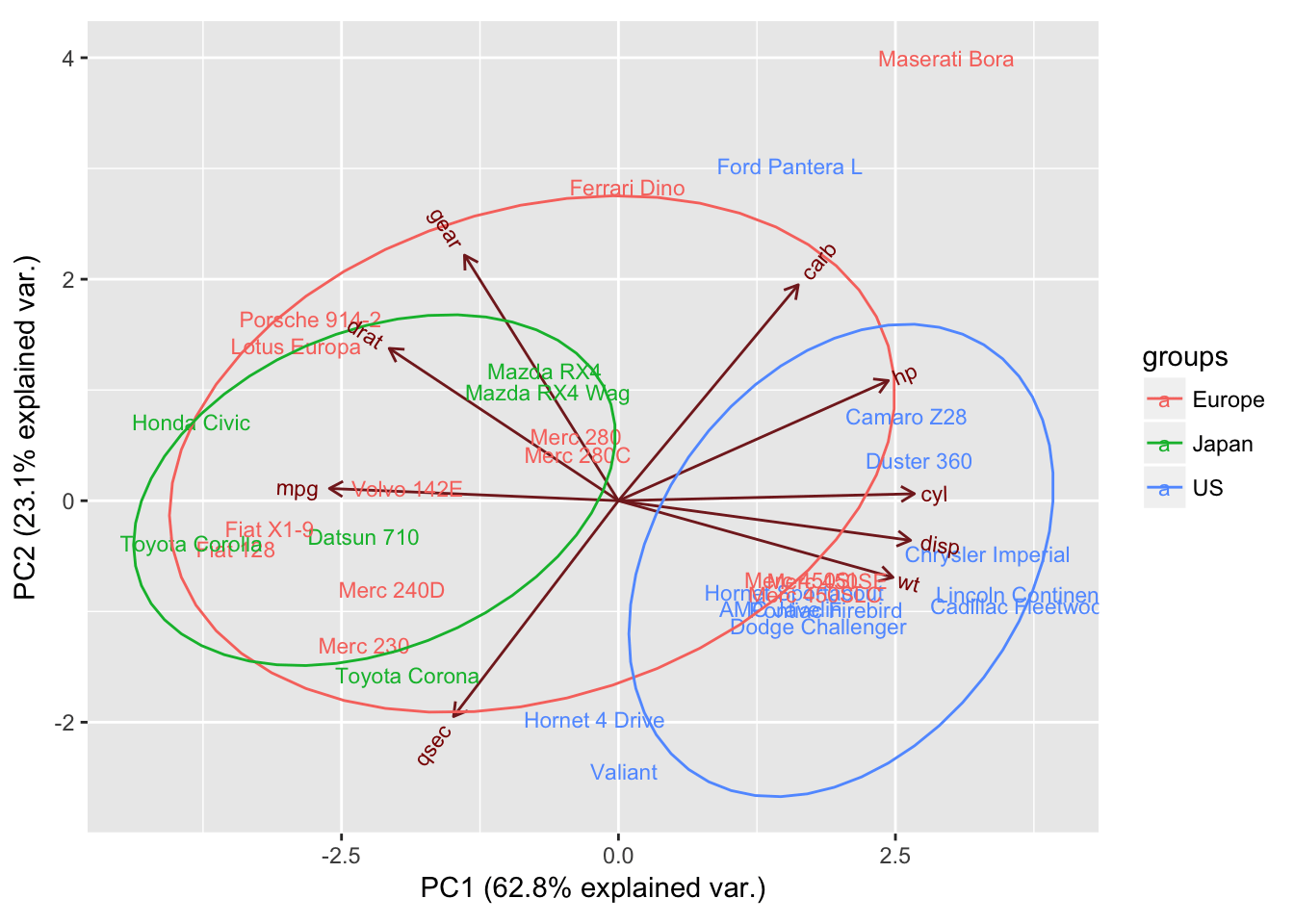You can also remove the arrows altogether, using var.axes. ggbiplot(mtcars.pca,ellipse=TRUE,obs.scale = 1, var.scale = 1,var.axes=FALSE, labels=rownames(mtcars), groups=mtcars.country)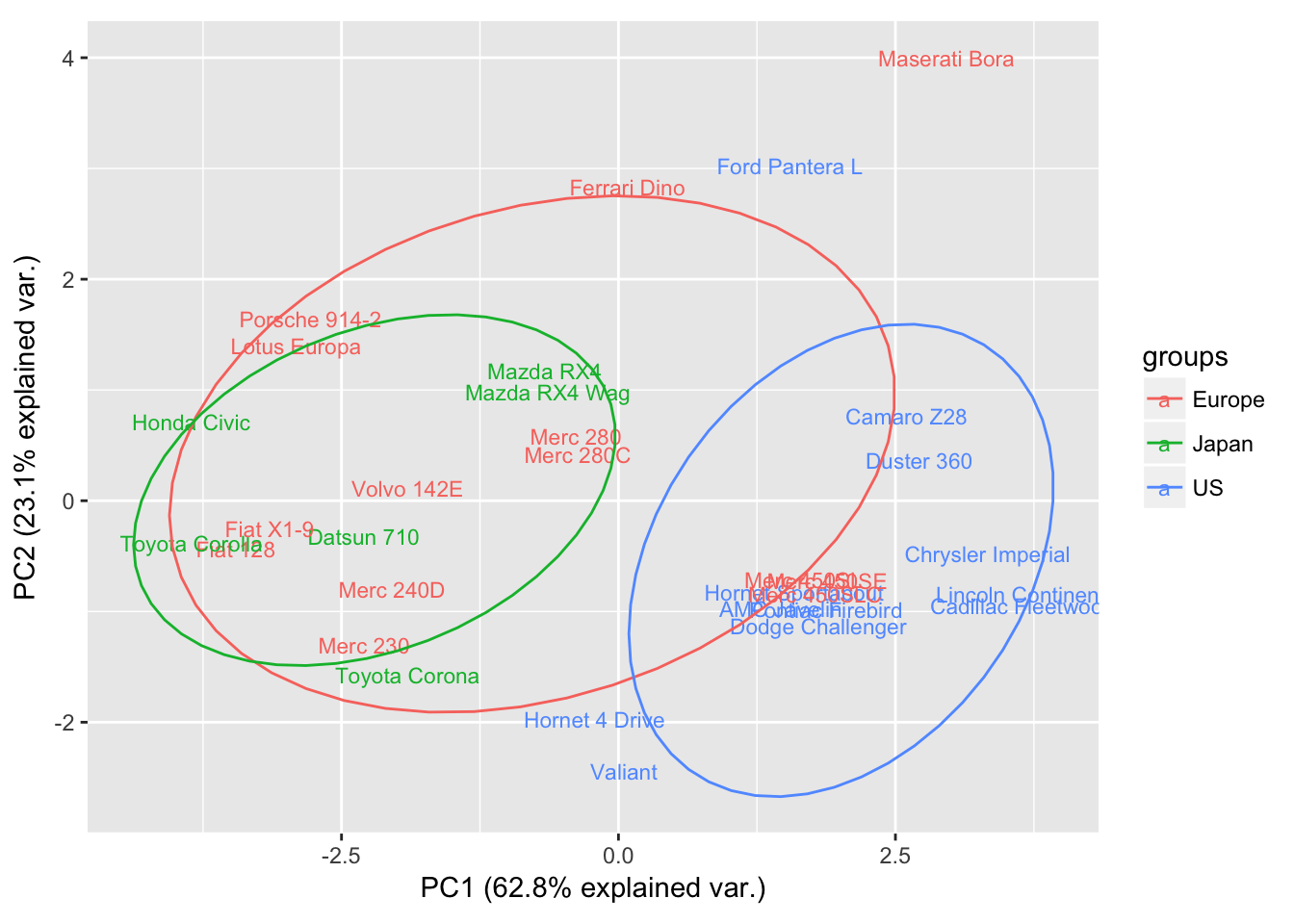## Customize ggbiplot As ggbiplot is based on the ggplot function, you can use the same set of graphical parameters to alter your biplots as you would for any ggplot. Here, you're going to: • Specify the colours to use for the groups with scale_colour_manual() • Add a title with ggtitle() • Specify the minimal() theme • Move the legend with theme() ggbiplot(mtcars.pca,ellipse=TRUE,obs.scale = 1, var.scale = 1, labels=rownames(mtcars), groups=mtcars.country) + scale_colour_manual(name="Origin", values= c("forest green", "red3", "dark blue"))+ ggtitle("PCA of mtcars dataset")+ theme_minimal()+ theme(legend.position = "bottom")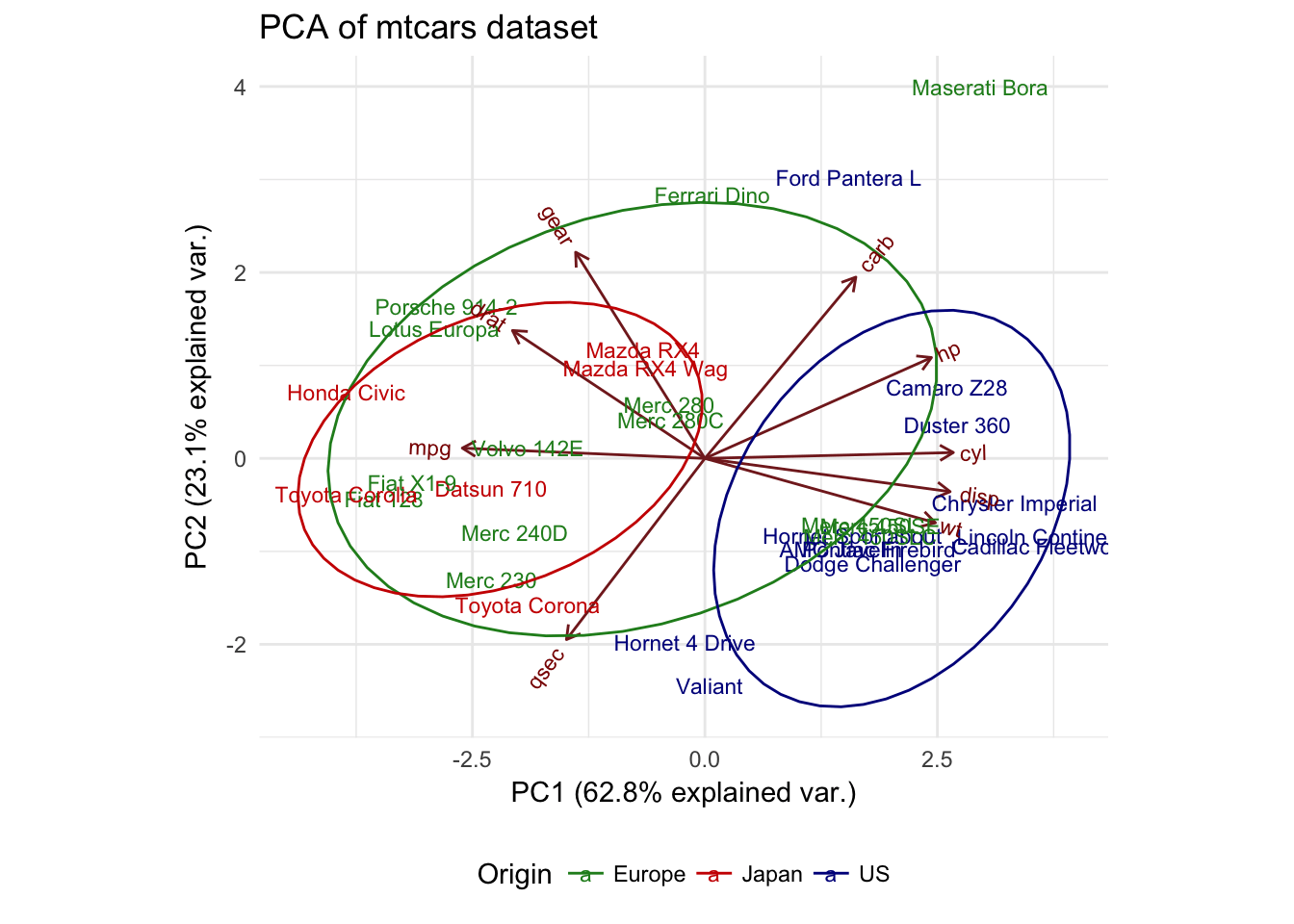## Adding a new sample Okay, so let's say you want to add a new sample to your dataset. This is a very special car, with stats unlike any other. It's super-powerful, has a 60-cylinder engine, amazing fuel economy, no gears and is very light. It's a "spacecar", from Jupiter. Can you add it to your existing dataset and see where it places in relation to the other cars? You will add it to mtcars, creating mtcarsplus, then repeat your analysis. You might expect to be able to see which region's cars it is most like. spacecar <- c(1000,60,50,500,0,0.5,2.5,0,1,0,0) mtcarsplus <- rbind(mtcars, spacecar) mtcars.countryplus <- c(mtcars.country, "Jupiter") mtcarsplus.pca <- prcomp(mtcarsplus[,c(1:7,10,11)], center = TRUE,scale. = TRUE) ggbiplot(mtcarsplus.pca, obs.scale = 1, var.scale = 1, ellipse = TRUE, circle = FALSE, var.axes=TRUE, labels=c(rownames(mtcars), "spacecar"), groups=mtcars.countryplus)+ scale_colour_manual(name="Origin", values= c("forest green", "red3", "violet", "dark blue"))+ ggtitle("PCA of mtcars dataset, with extra sample added")+ theme_minimal()+ theme(legend.position = "bottom")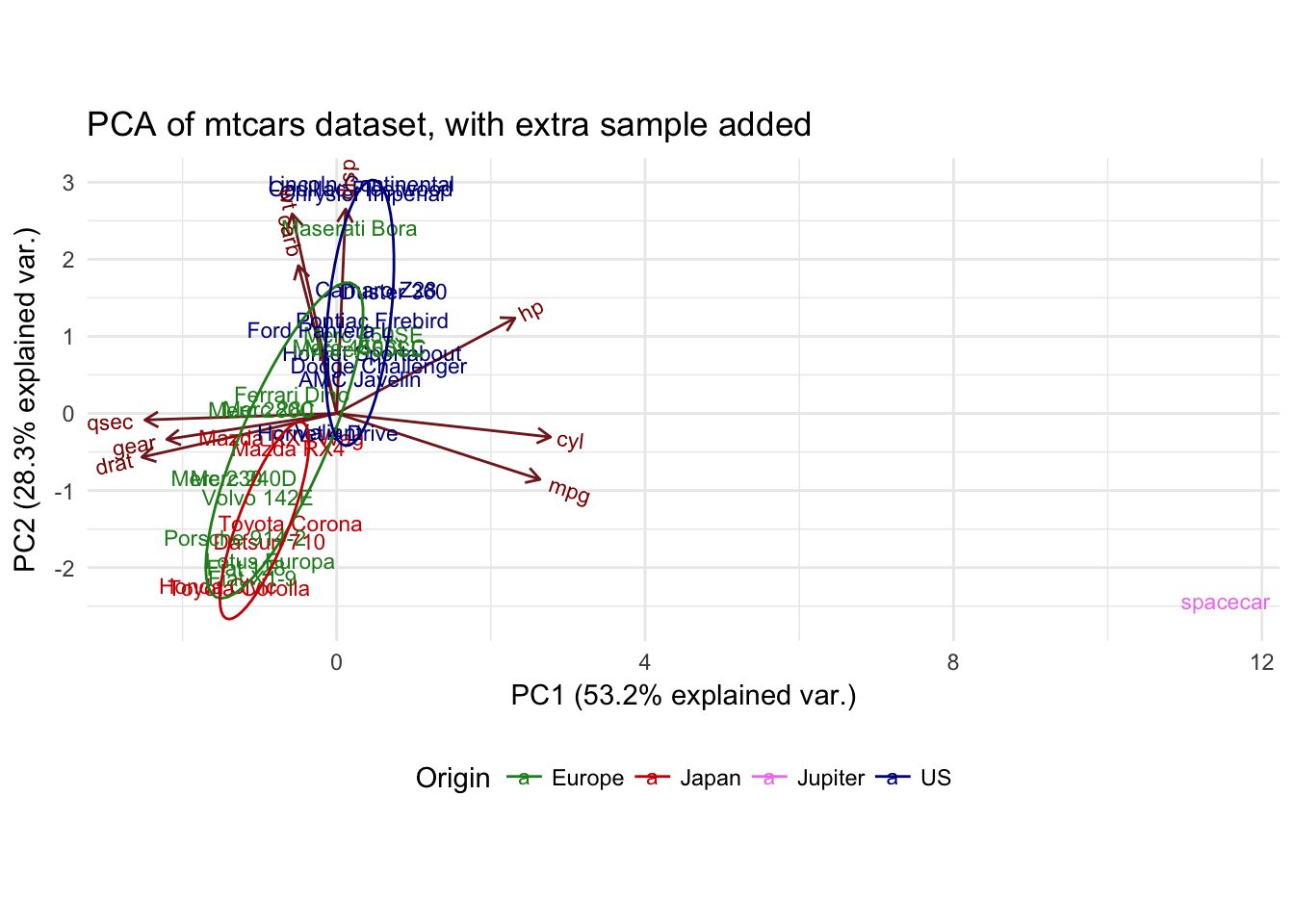But that would be a naive assumption! The shape of the PCA has changed drastically, with the addition of this sample. When you consider this result in a bit more detail, it actually makes perfect sense. In the original dataset, you had strong correlations between certain variables (for example, cyl and mpg), which contributed to PC1, separating your groups from one another along this axis. However, when you perform the PCA with the extra sample, the same correlations are not present, which warps the whole dataset. In this case, the effect is particularly strong because your extra sample is an extreme outlier in multiple respects. If you want to see how the new sample compares to the groups produced by the initial PCA, you need to project it onto that PCA. ## Project a new sample onto the original PCA What this means is that the principal components are defined without relation to your spacecar sample, then you compute where spacecar is placed in relation to the other samples by applying the transformations that your PCA has produced. You can think of this as, instead of getting the mean of all the samples and allowing spacecar to skew this mean, you get the mean of the rest of the samples and look at spacecar in relation to this. What this means is that you simply scale the values for spacecar in relation to the PCA's center (mtcars.pca$center). Then you apply the rotation of the PCA matrix to the spacecar sample. Then you can rbind() the projected values for spacecar to the rest of the pca$x matrix and pass this to ggbiplot as before: s.sc <- scale(t(spacecar[c(1:7,10,11)]), center= mtcars.pca$center)
s.pred <- s.sc %*% mtcars.pca$rotation mtcars.plusproj.pca <- mtcars.pca mtcars.plusproj.pca$x <- rbind(mtcars.plusproj.pca\$x, s.pred)

ggbiplot(mtcars.plusproj.pca, obs.scale = 1, var.scale = 1, ellipse = TRUE, circle = FALSE, var.axes=TRUE, labels=c(rownames(mtcars), "spacecar"), groups=mtcars.countryplus)+
scale_colour_manual(name="Origin", values= c("forest green", "red3", "violet", "dark blue"))+
ggtitle("PCA of mtcars dataset, with extra sample projected")+
theme_minimal()+
theme(legend.position = "bottom")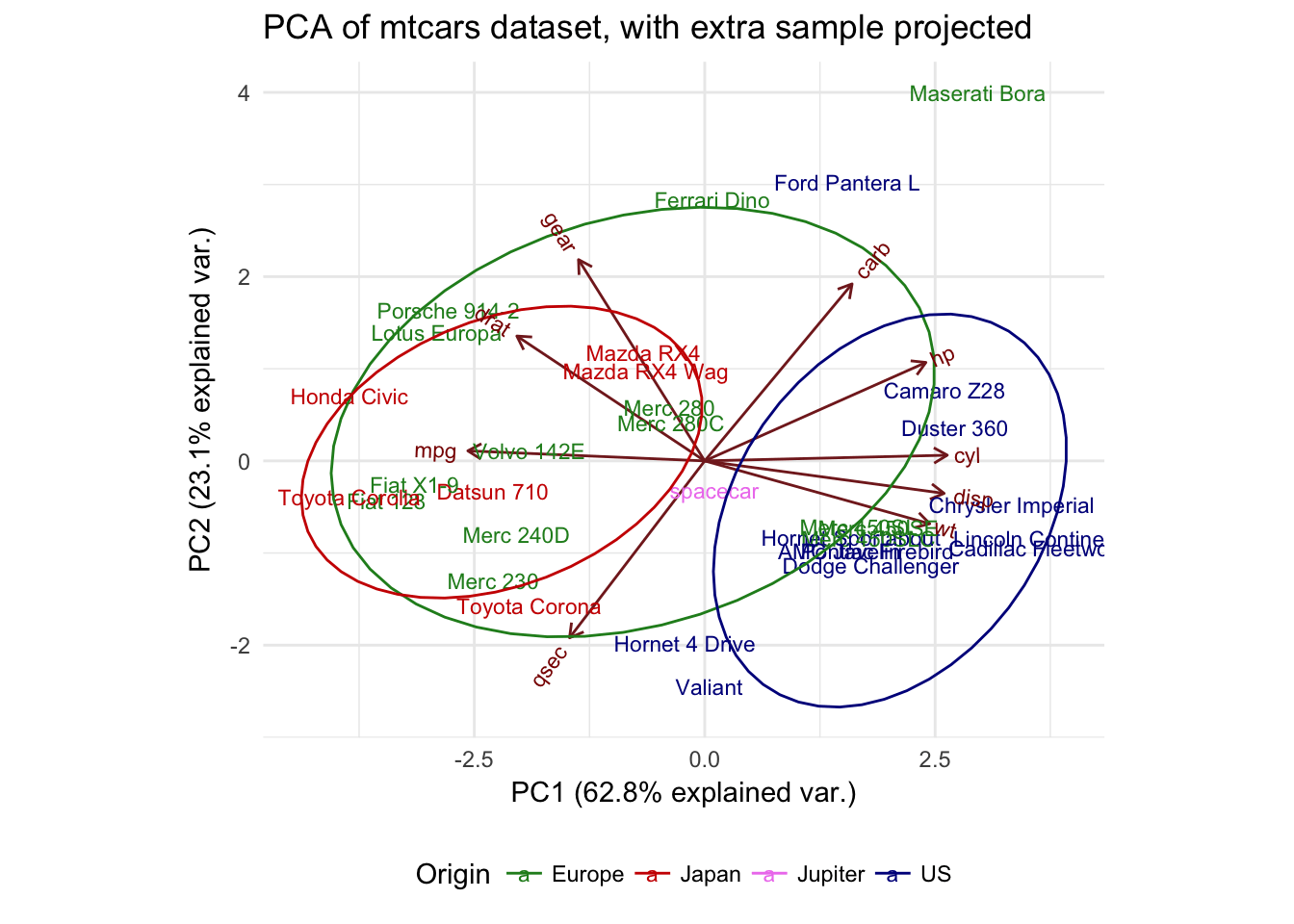This result is drastically different. Note that all the other samples are back in their initial positions, while spacecar is placed somewhat near the middle. Your extra sample is no longer skewing the overall distribution, but it can't be assigned to a particular group.

But which is better, the projection or the recomputation of the PCA?

It depends somewhat on the question that you are trying to answer; the recomputation shows that spacecar is an outlier, the projection tells you that you can't place it in one of the existing groups. Performing both approaches is often useful when doing exploratory data analysis by PCA. This type of exploratory analysis is often a good starting point before you dive more deeply into a dataset. Your PCAs tell you which variables separate American cars from others and that spacecar is an outlier in our dataset. A possible next step would be to see if these relationships hold true for other cars or to see how cars cluster by marque or by type (sports cars, 4WDs, etc).

## Wrap-up

So, there you have it!

You have learned the principles of PCA, how to create a biplot, how to fine-tune that plot and have seen two different methods for adding samples to a PCA analysis. Thanks for reading!